# Null space

## Null Space

In its simplest significance, the word null brings out the sense of canceling out, a sense of a void or emptiness, but how can we relate this to linear algebra and vector operations? Simple, this null definition we have on our heads will take us straight forward to the number zero, and so, in this case we will be looking into linear algebra operations, such as a homogeneous linear system which will return as a result the value of zero, in this case, the vector zero.

We start with a little review on concepts we have seen throughout the linear algebra chapters to remind us of what is a row or a column space of a matrix, and continue our practice on m by n matrix operations.

### Subspace linear algebra

We have already learned through the lesson on the properties of subspace that a subspace is a set, a collection of elements (these elements could be scalars or vectors, in our case we will use vectors) belonging to the real coordinate space (Rn) which fulfills the next three conditions:

1. The set (called S) contains the zero vector.
2. Closed under addition property: The addition of vectors found on the set produces a vector also in the set.
3. Closed under scalar multiplication property: If you multiply a constant to a vector in the set, the resultant vector is also part of the set.

And so, if all three conditions apply we say that the set S and a subspace:

To continue on the topic of subspace linear algebra and the operations or elements one can find in them, let us look at the components found in any given m by n matrix: First of all, always remember that "m by n matrix" refers to a matrix with m quantity of rows and n quantity of columns. For that, the concepts of row space and column space come about: we define row space as the full extent of rows in the given matrix, and the same goes for the column space which will denote the spread of the columns in the matrix including all of their linear combinations.

Going forward, an imperative operation to remember is matrix multiplication in which having two factors (each being a matrix) notice how the first factor (matrix on the left) must contain the same amount of columns as the amount of rows found in the second factor (the matrix on the right in the multiplication).

Matrix multiplication is shown clearly on our equation 2 below:

This little review is useful since we will work with matrices and multiplications (besides typical row reduction) while finding null space. So make sure you understand equation 2 before continuing into the next section.

### Null space of a matrix

After learning what is subspace, is time for us to focus on our main topic for today's lesson which is the null space. Let us start with the subspace definition, which tells us that in general a subspace is produced by a homogeneous linear system which can be geometrically represented on the real coordinate space passing through the origin.

And thus, the null space of a matrix A is the set of all the solutions given by the homogeneous system (homogeneous differential equation containing all the set of x's) which result in Ax=0. Here null space of A is denoted as N(A).

As mentioned before, the null space of a matrix A, or N(A), is a subspace of the real coordinate space (Rn) and this can be proved by verifying the three properties mentioned before in the first section of this lesson:

1. The zero vector can be found in N(A)
2. For each u and v in the set N(A), the sum of u+v is in N(A) (closed under addition)
3. For each u in the set N(A), the vector cu is in N(A). (closed under scalar multiplication)

To see if a vector u is in N(A) we simply multiply the matrix times the vector. If the product of A and u gives the zero vector, such as: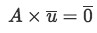Equation 3: Condition for vector u to be part of the null space of A

Something important to remember is that the result from the matrix product above is a vector with all of its components equal to zero. One may think this is just equal to zero and although technically this could be taken as correct, since its physical behaviour is the same, there is a greater significance behind the zero vector than just a zero number.

A zero vector will aid on our sense of dimension of null space, because although it doesn't point at any particular direction (you can think of it as just a dot), the zero vector will be able to denote how many dimensions in space are being taken as our reference frame for any given problem. For example: a zero vector such as (0,0) is not the same as a zero vector (0,0,0). The first one reflects a two dimensional problem (which means our results will rely on a plane), the second is a tri-dimensional problem (which means results come up in a tri-dimensional space such as the one we habitate in).

And so, a zero vector provides information about the physical characteristics of the system we are working with and the name "null space" then takes a much deeper meaning: null space is the result of a matrix being multiplied by a vector which results in components equal to zero, but still the "stage" of the problem remains. In other words, the computations you do with such matrices or vectors may cancel the values in them but the "stage" or frame of reference you were working on remains there, since it doesn't have a particular value, we just call it "null" instead of saying "is a void".

To continue, if we want to find a basis for the null space of a given matrix A, we have to follow the next general steps:

1. Solve for Ax=0.
1. In this case, you will be looking for the vector x and so the use of an augmented matrix will be needed.
2. In order to solve the augmented matrix you need to follow row-reduction methods.
3. The row reduction continues until you find the simplest correlation (or pivot points) between the components of vector x.
2. Write the general solution in parametric vector form.
3. The vectors you obtain are a basis for N(A)

*Note that the vectors in the basis are linearly independent.

In conclusion, we define null space of matrix A as the set of all vectors (or the subspace) which multiplied by the matrix A produce the zero vector as a result.

### How to find the null space of a matrix

Before we start working through examples on how to find null space for a given matrix, please make sure to have studied the lessons on representing a linear system as a matrix and linear independence, since these explain the basics on most of our operations for this lesson or the reasoning behind them.

#### Example 1

Is the vector u in the null space of matrix A?

• Having u and A as:

• For u to be in the null space of A, the condition A*u=0 needs to hold, and so we multiply the matrices following he process shown in equation 2:

And so, since we obtain a trivial solution (a zero vector) then vector u belongs to the null space of A.

This is one of the simplest examples about null space, which only requires you to find out if an already given vector is part of the null space for the given matrix. As long as you remember the condition found in equation 3 this should be a straightforward process.

We continue with one more example of this simple approach and then in examples 3 and 4 we go onto find the basis for null space of a given matrix.

#### Example 2

Is vector v in the null space of a matrix A as shown below?

• Once more, for v to be in the null space of A, the condition A*v=0 needs to hold. Let us multiply the matrices representing each vector to see if the final solution is trivial. Thus, having:

• We multiply:

Since the resulting vector is not a zero vector, this means vector v does not belong to the null space in A.

#### Example 3

Find a basis for the null space of A:

• In this case we need to find a vector that multiplied for A will produce the condition A*x=0, such as:

• In order to find that vector x, we follow the steps listed in the last part of the past section on this lesson by using an augmented matrix and matrix-reduction to solve Ax=0 and find the set of three pivots, one for each component of x. Remember that you can always go back to the lesson on row reduction if you have doubts on how to reduce the matrix.

• And so we write the resultant vector in parametric form to deliver our final answer:

#### Example 4

Find a basis for the null space of A:

• We find the vector that will satisfy the condition A*x=0, reducing the augmented matrix:

• And so:

• We write the general solution in parametric vector form:

• And thus, the final solution says that the basis for null space of matrix A is:

As you can see the basis of a matrix for null space, such as the ones found throughout these examples, reiterate the conclusion from our last section on this lesson: null space will be the vectors (in this case set of all vectors) which will result in the zero vector when they are multiplied by the given matrix.

Notice that we have done all these multiplications by keeping the matrix as the first factor (factor on the left), but another interesting look at our topic of today comes from the computation of the right and left null spaces which depend on the order in which the vector set is multiplied to the matrix. This comes from the fact that matrix multiplication is not commutative (the order of the factors DOES alter the product in matrix multiplication) and so, the right null space is not the same as the left null space for a given matrix.

To finalize our lesson, we recommend this book excerpt on null space, column space and row space since it contains not only well explained concepts but thorough examples.

##### Do better in math today
6.
Subspace of $\Bbb{R}^n$
6.1
Properties of subspace
6.2
Column space
6.3
Null space
6.4
Dimension and rank

### Null space

#### Lessons

The null space of a matrix $A$ is the set $N(A)$ of all solutions of the homogeneous equation $Ax=0$

The null space of a matrix $A$ is a subspace of $\Bbb{R}^n$. The first question shows the proof of this.

To see if a vector $u$ is in $N(A)$ (nullspace of $A$), we simply compute:

$Au=0$

If the product of $A$ and $u$ gives the zero vector, then it is in the null space of $A$.

To find a basis for the null space of A, we have to:
1) Solve for $Ax=0$.
2) Write the general solution in parametric vector form.
3) The vectors you see is a basis for $N(A)$.

Note that the vectors in the basis are linearly independent.
• Introduction
Null Space Overview:
a)
Definition of the Null space
$N(A) =$ null space
• A set of all vectors which satisfy the solution $Ax=0$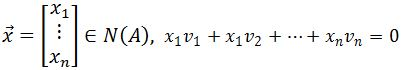b)
A vector u in the null space
• Multiply matrix $A$ and vector $u$
$Au=0$ means $u$ is in the null space

c)
Finding a basis for the null space
• Solve for $Ax=0$
• Write the general solution in parametric vector form
• The vectors you see in parametric vector form are the basis of $N(A)$.

• 1.
Showing that the null space of $A$ is a subspace
Show that $N(A)$ (null space of $A$) is a subspace by verifying the 3 properties:
1) The zero vector is in $N(A)$
2) For each $u$ and $v$ in the set $N(A)$, the sum of $u+v$ is in $N(A)$ (closed under addition)
3) For each $u$ in the set $N(A)$, the vector $cu$ is in $N(A)$. (closed under scalar multiplication)

• 2.
Verifying a vector is in the null space
Is the vector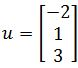in the null space of the matrix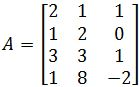• 3.
Is the vector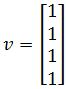in the null space of the matrix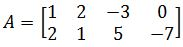• 4.
Finding a basis for the null space
Find a basis for the null space of $A$ if: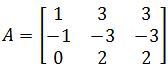• 5.
Find a basis for the null space of $A$ if: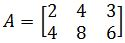##### Do better in math today
6.
Subspace of $\Bbb{R}^n$
6.1
Properties of subspace
6.2
Column space
6.3
Null space
6.4
Dimension and rank